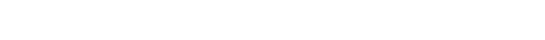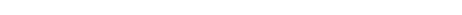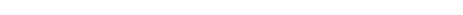A dummy variable is a variable that takes values of 0 and 1, where the values indicate the presence or absence of something (e.g., a 0 may indicate a placebo and 1 may indicate a drug). Where a categorical variable has more than two categories, it can be represented by a set of dummy variables, with one variable for each category. Numeric variables can also be dummy coded to explore nonlinear effects. Dummy variables are also known as indicator variables, design variables, contrasts, one-hot coding, and binary basis variables.

## Example

The table below shows a categorical variable that takes on three unique values: A, B, and C. The three dummy variables that represent this variable are shown to the right, where each variable takes a value of 0 when its category is not present, and a value of 1 when its category is present.

A100
A100
B010
A100
B010
C001
A100

## The role of dummy variables in analysis

Dummy variables are the main way that categorical variables are included as predictors in statistical and machine learning models.  For example, the output below is from a linear regression where the outcome variable is profitability, and the predictor is the number of employees. With statistical models such as linear regression, one of the dummy variables needs to be excluded (by convention, the first or the last), otherwise the predictor variables are perfectly correlated; in the example below, the variable representing companies with a single employee (the owner) has been excluded.This is expressed as the following formula:As the variables are all dummy variables, this means that they have values of 0 and 1.  The predicted profit for a firm with one employee is then:For a firm with three employees it is:We are multiplying  by 1, as it the parameter for the dummy variable which can only take values of 0 and 1 (i.e., we do not multiply by the number of employees).

### Alternatives to dummy variables

The main benefit of dummy variables is that they are simple. There are often better alternative basis functions, such as orthogonal polynomials, effects coding, and splines.